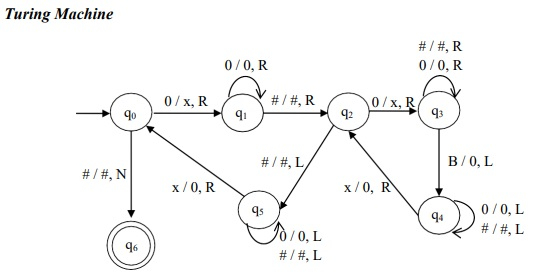# Construct a TM performing multiplication of two unary numbers

Data Structure AlgorithmsComputer ScienceComputers

## Algorithm

Step 1 - Read the leftmost ‘0’ replace it by ‘x’ and move right to process the immediate symbol after ‘#’.

Step 2 - Replace the symbol ‘0’ by x and move right reach the first ‘B’ after ‘#’

Step 3 - Replace B by ‘0’ and move left until the nearest ‘x’ is reached

Step 4 - Replace the ‘x’ by 0 and move right to process the next symbol of the multiplicand.

Step 5 - Perform steps 2, 3 and 4 until all the symbols of the multiplicand are processed.

Step 6 - Move left to replace the symbol of the multiplier, ‘x’ by ’0’.

Step 7 - Perform steps 1 to 6 until all the symbols of the multiplier are processed.

## Turing Machine

The Turing Machine (TM) is as followsThe Turing machine, M is given by

M = (Q, Σ, Γ, δ, q0, B, F)

Where,

• Q = {q0, q1, q2, q3, q4, q5, q6}

• Σ = {0, #}

• Γ = {0, #, x, B}

• q0 = {q0}

• B = {B}

• F = {q6}

• δ ⇒ given by the above transition diagram.